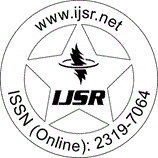International Journal of Science and Research (IJSR)
Call for Papers | Fully Refereed | Open Access | Double Blind Peer Reviewed

Research Paper | Mathematics | India | Volume 3 Issue 11, November 2014

# On The Non Homogeneous Heptic Equation with Five Unknowns

P. Jayakumar  | K. Sangeetha 

Abstract: The non homogeneous Diophantine equation of degree seven with five unknowns represented by (x^2-y^2) (9x^2+9y^2-16xy) =21 (X^2-Y^2) z^5 is analyzed for its non - zero distinct integer solutions. Employing suitable linear transformations and applying the method of cross multiplication, four different patterns of non-zero distinct integer solutions to the heptic equation under consideration are obtained. A few interesting relation between the solutions and special numbers namely Polygonal numbers, Pyramidal numbers, Centered Pyramidal numbers, Star numbers and Stella octangular numbers are exhibited.

Keywords: The non homogeneous Diophantine equation, Heptic equation with five unknowns, integral solutions, special numbers, a few interesting relation,

Edition: Volume 3 Issue 11, November 2014,

Pages: 3156 - 3162